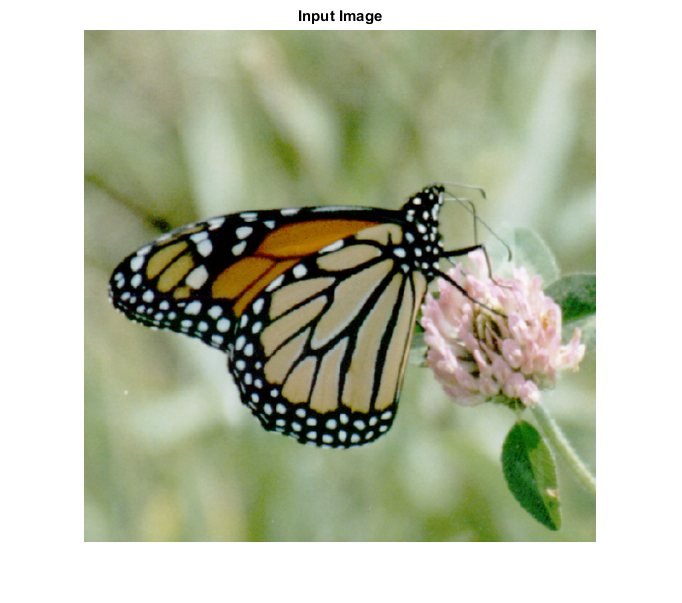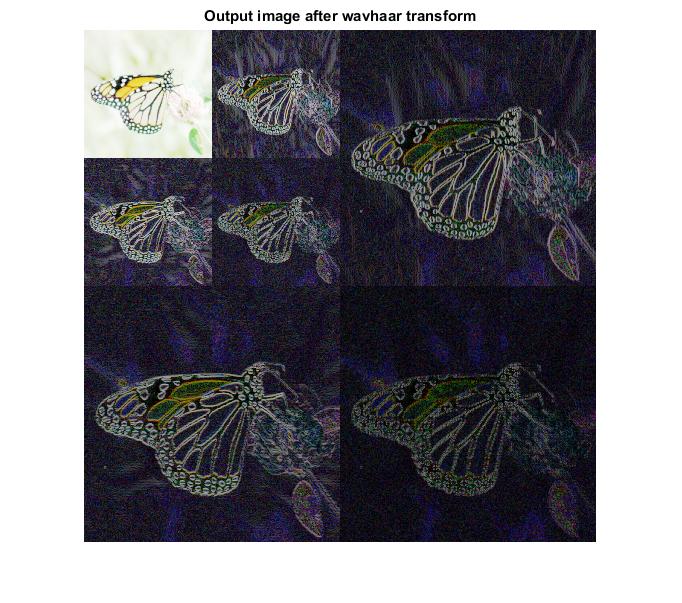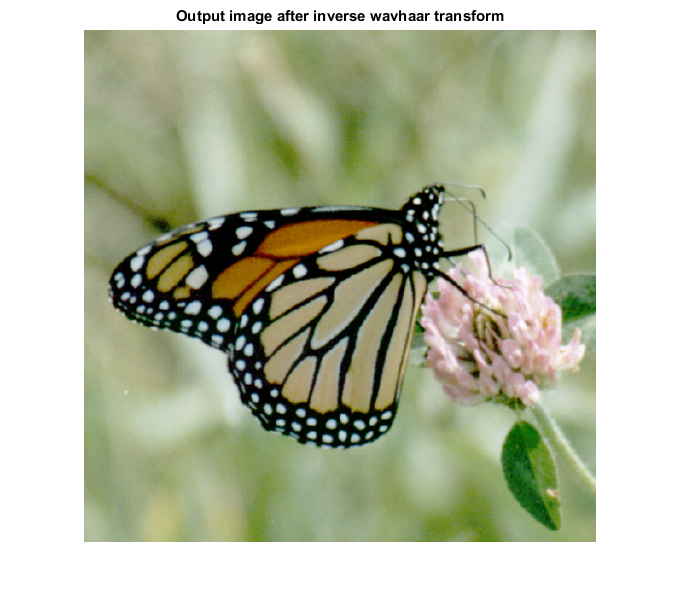# IWAVHAAR_CVIP

iwavhaar_cvip() - performs inverse wavelet transform based on Haar wavelet.

## SYNTAX

`[ out_mat ] = iwavhaar_cvip( img,dec )`

Input Parameters Include:

• img - The orignial image spectrum.
• dec - The decomposition level. Same as the level used for forward transform. An integer greater than or equal to 1.

Output Parameter Include:

• out_mat - The wavelet transform of the image using Haar wavelet transform.

## DESCRIPTION

This function computes the inverse wavelet transform of the input image based on the Haar wavelet transform. The argument block_size should match the same value given for the forward transform. For more details look at the reference below.

## REFERENCE

1. Scott E Umbaugh. DIGITAL IMAGE PROCESSING AND ANALYSIS: Applications with MATLAB and CVIPtools, 3rd Edition.

## EXAMPLE

```%Read image

% b is of type double, so it requires hist_stretch_cvip for remapping.

b = double(a);

% Decomposition level

dec = 2;

% Calling wavhaar transform

w = wavhaar_cvip(b,dec);

% This is a good way to remap

S = log(1+abs(w));

S = remap_cvip(S);

% Calling inverse transform

out_mat= iwavhaar_cvip(w,dec);

% Display input image

figure;imshow(a);title('Input Image');

% Display Output images

figure, imshow(S,[]);title('Output image after wavhaar transform')  % tranforms for display.

figure; imshow(hist_stretch_cvip(out_mat,0,1,0,0),[]);title('Output image after inverse wavhaar transform');
```